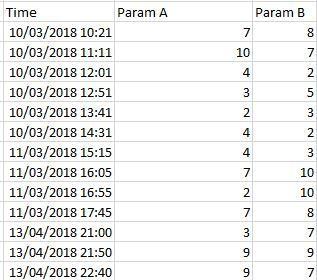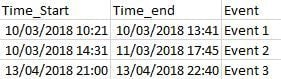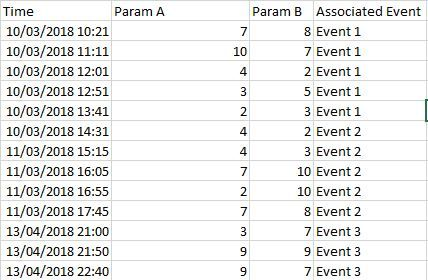# Classification Time.Dataset1 with respect to Time ranges from Dataset2

Member Posts: 2Contributor I
edited August 2019 in Help

Hello, I am Gianfranco, I am new. I am Post-DOC in the field of Industrial. First of all, thanks for sharing your knowledge. You saved me for several nights J. Chemistry/Heterogeneous Catalysis.  Now, my problem is: I have two datasets (DATASET1 and DATASET2).

DATASET1 looks like:DATASET2I'd like to match the Time in DATASET1 to the Time ranges reported in DATASET2, like:In excel is very easy (index and match functions), but slow.

Gianfranco

Tagged:

• Moderator, RapidMiner Certified Analyst, Member, University Professor Posts: 568Unicorn

Hi Gianfranco,

Yes. It's actually simpler on RapidMiner. You can use the Join operator. Here is a test.

`<?xml version="1.0" encoding="UTF-8"?><process version="9.0.002">  <context>    <input/>    <output/>    <macros/>  </context>  <operator activated="true" class="process" compatibility="9.0.002" expanded="true" name="Process">    <process expanded="true">      <operator activated="true" class="read_excel" compatibility="9.0.002" expanded="true" height="68" name="Read HTA" width="90" x="45" y="34">        <parameter key="excel_file" value="/Users/rodrigo/data.xlsx"/>        <parameter key="sheet_number" value="2"/>        <list key="annotations"/>        <parameter key="date_format" value="MMM d, yyyy h:mm:ss a z"/>        <list key="data_set_meta_data_information">          <parameter key="0" value="Date.true.date_time.attribute"/>          <parameter key="1" value="Systolic.true.integer.attribute"/>          <parameter key="2" value="Diastolic.true.integer.attribute"/>        </list>        <parameter key="read_not_matching_values_as_missings" value="false"/>      </operator>      <operator activated="true" class="read_excel" compatibility="9.0.002" expanded="true" height="68" name="Read Pulse" width="90" x="45" y="136">        <parameter key="excel_file" value="/Users/rodrigo/data.xlsx"/>        <list key="annotations"/>        <parameter key="date_format" value="MMM d, yyyy h:mm:ss a z"/>        <list key="data_set_meta_data_information">          <parameter key="0" value="Date.true.date_time.attribute"/>          <parameter key="1" value="Pulse.true.integer.attribute"/>        </list>        <parameter key="read_not_matching_values_as_missings" value="false"/>      </operator>      <operator activated="true" class="concurrency:join" compatibility="9.0.002" expanded="true" height="82" name="Join" width="90" x="179" y="85">        <list key="key_attributes">          <parameter key="Date" value="Date"/>        </list>      </operator>      <connect from_op="Read HTA" from_port="output" to_op="Join" to_port="left"/>      <connect from_op="Read Pulse" from_port="output" to_op="Join" to_port="right"/>      <connect from_op="Join" from_port="join" to_port="result 1"/>      <portSpacing port="source_input 1" spacing="0"/>      <portSpacing port="sink_result 1" spacing="0"/>      <portSpacing port="sink_result 2" spacing="0"/>    </process>  </operator></process>`

I wanted to attach my data.xlsx file but it doesn't let me, so here are pics:This is my average pulse.This is my average systolic and diastolic blood pressure.

(Don't be scared, it's my cardiac monitor information and I have been doing some workout on the afternoon, so blood pressure is expected to be higher than normal).

The process looks like this:You should make sure you don't use id attribute as key, and then add your date attributes to the list.

All the best,

Rodrigo.

• Member Posts: 2Contributor I

Hello, thanks for your nice reply. However, in my case the problem is that there the TIME attribute is not a unique identifier (ID) between dataset1 and dataset2. Instead, in dataset2 I report that, e.g.

between 10/03/18 10:21 and 10/03/18 13:41 is Event 1

In dataset 1, however,  between the reported times there are 5 examples at 10:21, 11:11, 12:01, 12:51 and 13:41 which should be labeled as EVENT 1.

This means that,

IF (Dataset1.Time >=10:21 (from dataset2) && Dataset1.Time<=13:41 (from dataset2))

THEN this event is classified as EVENT1,

ELSEIF Dataset1.Time >=14:31 (from dataset2) && Dataset1.Time>17:45 (from dataset2)).....

THEN Event 2

and so on..

How could I do something like this, whithout using the if else construction, for a database of events which might change?